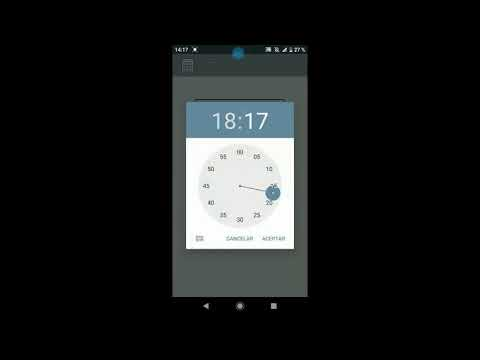# Hour Calculator for Work - Time Calc - Salary CalcEveryone
37Hour Calculator for work: Job Hours Tracker - Salary Calculator- Time Calculator

Add,subtract,multiply or divide hours and minutes. Useful to calculate the job hours, salary or which work with better salary to choose.

Hour Calculator for work: Job Hours Tracker - Salary Calculator- Time Calculator: Calculate time difference between times. Store the records by date, calculate the total by days, weeks, months, hours and minutes.

Examples of this Hour Calculator for work: Job Hours Tracker - Salary Calculator- Time Calculator:

Hours and Minutes entry: 07:02
Hours and Minutes exit: 16:58
Time Calc Hour Calculator for work: 09:56
Price Hour: 11.57
Salary: 114.93

Hours and Minutes entry: 07:55
Hours and Minutes exit: 15:14
Time Calc Hour Calculator for work: 07:19
Price Hour: 9.9
Salary: 72.44

Controlling overtime in your work will be easier with this Hour Calculator for work: Job Hours Tracker - Salary Calculator- Time Calculator. You will be able to know the hours worked and the salary.

You can perform your calculations in hours, minutes or in decimal, assign them a price and calculate the salary.

Use the Hour Calculator for work: Job Hours Tracker - Salary Calculator- Time Calculator to set an hour of entry and exit, the Hour Calculator for work: Time Calculator - Salary Calculator- Job Hours Tracker will calculate it for you.

Perfect for workers to calculate in their work the Salary or Time Calc. With this Hour Calculator for work: Job Hours Tracker - Salary Calculator- Time Calculator you can calculate your salary easily.

This Hour Calculator for work: Job Hours Tracker - Salary Calculator- Time Calculator will also help us Time Calc between minutes and hours. See some example of calculations of hours and minutes:

Hour Calculator for work: Job Hours Tracker - Salary Calculator- Time Calculator:

Time Calc: 69 minutes +82 minutes +3:03 hours = 5:34 hours
Time Calc: 100 minutes +5:42 hours +72 minutes = 8:34 hours

It works well for part-time jobs and know at the end of the day the total hours worked and salary. We multiply it by the hour of work price and we will obtain the salary of the work.

Hour Calculator for work: Job Hours Tracker - Salary Calculator- Time Calculator: Do you know how many hours are 724 minutes? Time Calc: It's 12:04 hours. With this hour and minute calculator - Time Calc it is very easy. Do not waste time with calculations of complex hours, with this Hour Calculator for work: Job Hours Tracker - Salary Calculator- Time Calculator is easy.

It is useful to calculate hours or Time Calc in companies with many employees, we calculate the hours of an employee and know his salary. Do you have many hourly employees and don't know what salary they have? With this Hour Calculator for work: Job Hours Tracker - Salary Calculator- Time Calculator you will have it controlled.

This Hour Calculator for work: Job Hours Tracker - Salary Calculator- Time Calculator has many uses:

-Hour Calculator: Calculate Cumulative study hours.
-Salary calculator for my workers
-Hour Calculator: Calculate Hours I've lost in traffic jams.
-Hour Calculator that accumulate breaks at work.
-Hour Calculator: Calculate Hours and minutes of exercise per week.
-Salary calculator: Calculate the salary or salary of your different jobs.
-Hour Calculator: Calculate Hours and minutes I do in my work for hours.
-Salary calculator in my different jobs by hours.
-Hour Calculator: How long does it take to make a project.

With this Hour Calculator for work: Job Hours Tracker - Salary Calculator- Time Calculator you will not need to waste a minute in making all these difficult time calculations.

Try this Hour Calculator for work: Job Hours Tracker - Salary Calculator- Time Calculator make us a comment and we will improve this hours and minutes calculator for you!

Thank you for using this Hour Calculator for work: Job Hours Tracker - Salary Calculator- Time Calculator!
Collapse

Review Policy
5.0
37 total
5
4
3
2
1

## What's New

Bug fixes We add functionalities to the calculator hours and minutes.
Collapse

Updated
January 5, 2020
Size
3.2M
Installs
1,000+
Current Version
1.05
Requires Android
4.4 and up
Content Rating
Everyone
Permissions
Offered By
TrasCo Studios